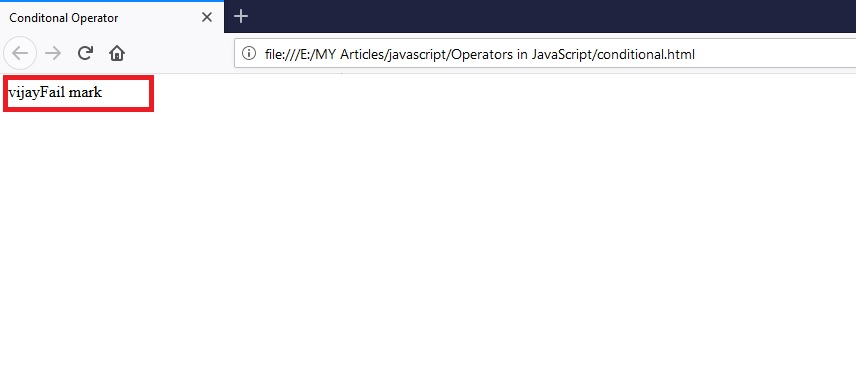# Operators in JavaScript

## Introduction

In the previous chapter, we learned about Variables in JavaScript, its scope, and how to use variables in JavaScript with example programs.

In this chapter, we will learn about operators in JavaScript. Many programming languages use operators, and every programming language has an operator. Without further ado, let's take a look at the operators in JavaScript.

## What is an Operator?

The operator is a symbol that performs some function in one or more values or functions. JavaScript operators perform math or logical functions in a variable. JavaScript supports the following types of operators:
• Arithmetic Operators
• Relational or comparison Operators
• Logical Operators
• Bitwise Operators
• Assignment Operators
• Conditional or ternary Operators

## Arithmetic Operators

These operators are used to perform arithmetic operations. Arithmetic operators are of two types:
• Unary Operators – It will operate a single operand
• Binary Operators – It will operate two operands
 Arithmetic Operators Description Binary Operators + It performs an addition operation - It performs a subtraction operation * It performs a multiplication operation / It performs a division operation % It calculates the remainder after division Unary Operators ++ It performs an increment on a given number -- It performs a decrement on a given number

Example of Binary Operators

Try it yourself:
1. <!DOCTYPE html>
2. <html>
4.     <meta charset="utf-8">
5.     <title>Arithmetic Operators</title>
7. <body>
8.     <h2>Arithmetic Operators in JavaScript</h2>
9.     <script type="text/javascript">
10.         var a=10;
11.         var b=20;
12.         document.write("The sum of two numbers a=10, b=20 <br>");
14.         document.write("<br>");
15.         document.write("Subraction :"+(a-b)); //subraction
16.         document.write("<br>");
17.         document.write("Multiplication :"+(a*b)); //Multiplication
18.         document.write("<br>");
19.         document.write("Division :"+(a/b)); //Division
20.         document.write("<br>");
21.         document.write("Modules :"+(a%b)); // Moudulus calculate the reminder
22.         document.write("<br>");
23.     </script>
24. </body>
25. </html>
Output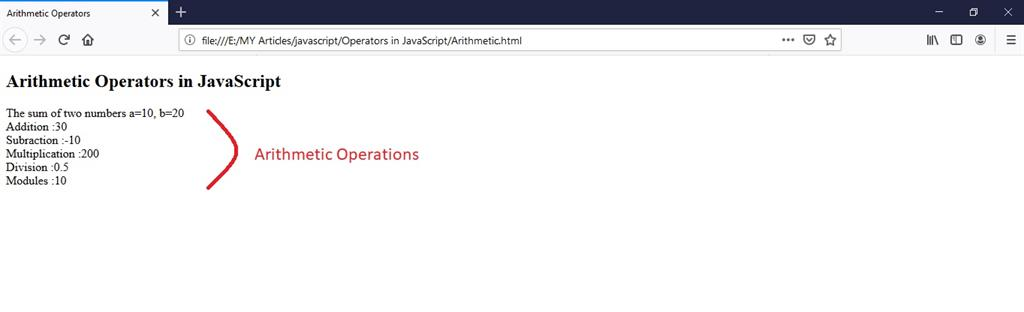Example of Unary Operators

Try it yourself:
1. <!DOCTYPE html>
2. <html>
4.     <meta charset="utf-8">
5.     <title>Urinary Operators</title>
7. <body>
8.     <h2>Urinary Operators in JavaScript</h2>
9.     <script type="text/javascript">
10.         var a=20;
11.         var b=30;
12.         document.write("The sum of two numbers a=20, b=30 <br>");
13.         //post increment
14.         document.write("Post increment 20++ :"+(a++));
15.         document.write("<br>");
16.         document.write("After increment the value of a is :"+(a));
17.         document.write("<br>");
18.         //pre increment
19.         document.write("Pre increment ++30 :"+(++b));
20.         document.write("<br>");
21.         document.write("After increment the value of b is :"+(b));
22.         document.write("<br> <br>");
23.         //post decrement
24.         document.write("Decrement Operators <br>");
25.         document.write("Post decrement 20-- :"+(a--));
26.         document.write("<br>");
27.         document.write("After decrement the value of a is :"+(a));
28.         document.write("<br>");
29.         //pre decrement
30.         document.write("Pre decrement --30 :"+(--b));
31.         document.write("<br>");
32.         document.write("After decrement the value of b is :"+(b));
33.     </script>
34. </body>
35. </html>
Output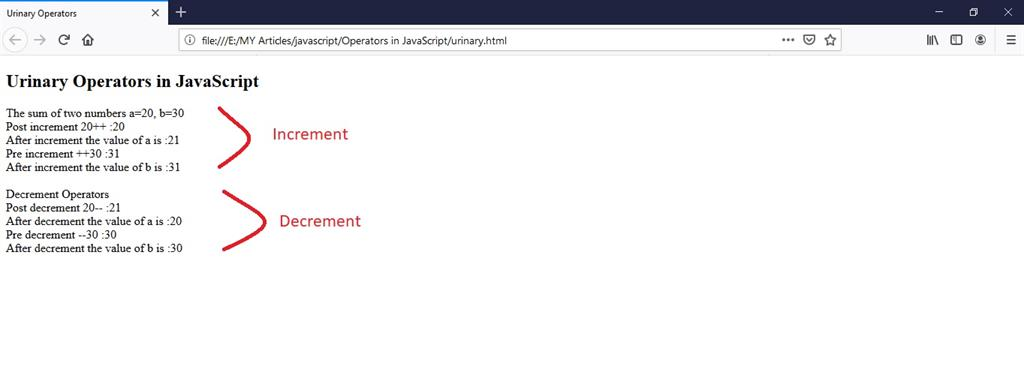String using ‘+’ Operator

Try it yourself:
1. <!DOCTYPE html>
2. <html>
4.     <title>String Operator</title>
6. <body>
7.     <h2>String Operator in JavaScript</h2>
8.     <script type="text/javascript">
9.         var str = "Hi"//string
10.         var str1 = "C sharp cornner!";
11.         document.write(str+str1); //string operator
12.     </script>
13. </body>
14. </html>
Output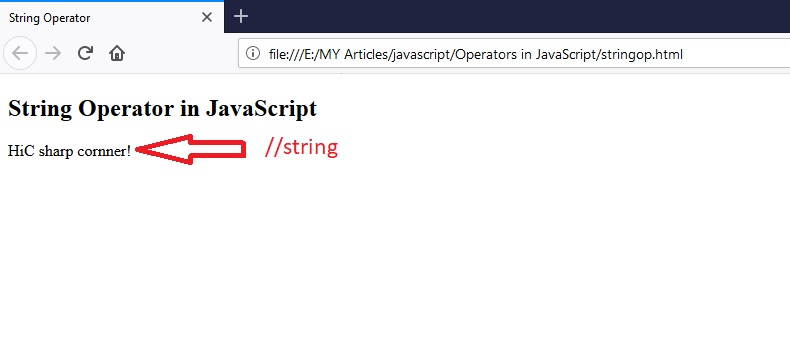## Relational or Comparison Operators

These operators are used to compare two values or variables. They are used to find the relationship between two values or compare the relationship between two values that yield the result true or false.

 Comparison operator Description == Equal to === Equal value and Equal type >= Greater than equal to <= Less than equal to > Greater than < Less than != Not equal to !== Not equal value and not equal type

Example of a comparison operator

Try it yourself:
1. <!DOCTYPE html>
2. <html>
4.     <title>Comparison Operator</title>
6. <body>
7.     <h2>Relational or Comparison Operators</h2>
8.     <script type="text/javascript">
9.         var a=5;
10.         var b=7;
11.         document.write(a==b);
12.         document.write("<br>");
13.         document.write(a===b);
14.         document.write("<br>");
15.         document.write(a>=b);
16.         document.write("<br>");
17.         document.write(a<=b);
18.         document.write("<br>");
19.         document.write(a>b);
20.         document.write("<br>");
21.         document.write(a<b);
22.         document.write("<br>");
23.         document.write(a!=b);
24.         document.write("<br>");
25.         document.write(a!==b);
26.         document.write("<br>");
27.     </script>
28. </body>
29. </html>
Output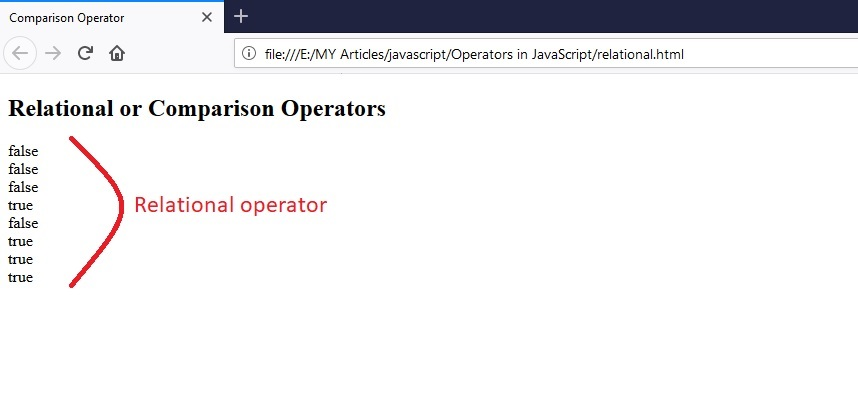## Logical Operator

These operators are used to perform Boolean expressions. This is true or false. This makes a logical AND, OR, and NOT. It is often used for conditional statements.

 Logical Operator Description && It performs logical AND operation || It performs logical OR operation ! It performs logical Not operation

Example of a logical operator

Try it yourself:
1. <!DOCTYPE html>
2. <html>
4.     <meta charset="utf-8">
5.     <title>Logical Operator</title>
6.     <script type="text/javascript">
7.         function verify() {
8.             var name = document.getElementById("nam").value;
9.             var pass = document.getElementById("pwd").value;
10.             if ((name=="vijay") && (pass=="logical")) //user name and password
11.             {
13.             }
14.             else
15.             {
17.
18.             }
19.         }
20.     </script>
22. <body>
23.     <h2>Logigal operator in JavaScript</h2>
25.     User Name<input type="text" name="name" id="nam" placeholder="Enter your Name">
26.     <br><br>
28.     <br><br>
29.     <input type="submit" value="submit" onclick="verify();">
30. </body>
31. </html>
Output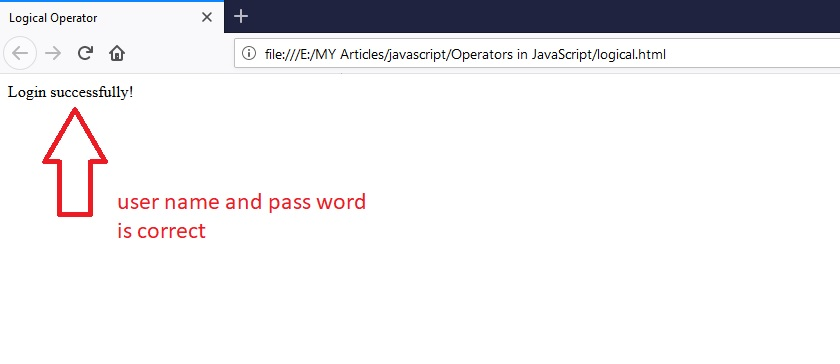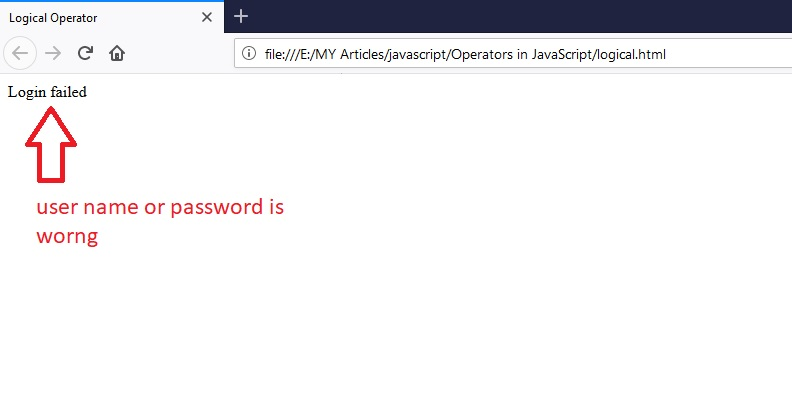## Bitwise Operators

JavaScript bitwise operators are used to perform functions on their bits. Bit means (0 and 1s) or true or false. The sequence of 8 bits is called byte. The bitwise operator converts a given decimal number into a binary number.

 Bitwise operators Description & Performs AND operation on the bits of operands | Performs OR operation on the bits of operands ^ Performs XOR operation on the bits of operands ~ Performs NOT operation on the bits of operands << Left shift is multiplication >> Right Shift is division >>> Right Shift with zero

Example of a Bitwise operator

Try it yourself:
1. <!DOCTYPE html>
2. <html>
4.     <title>Bitwise Operator</title>
6. <body>
7.     <h2>Bitwise Operator in JavaScript</h2>
8.     <script type="text/javascript">
9.         document.write("AND Operator (1 & 1) is :"+(1 & 1));
10.         document.write("<br>");
11.         document.write("AND Operator (1 & 0) is :"+(1 & 0));
12.         document.write("<br>");
13.         document.write("OR Operator (0 | 0) is :"+(0 | 0));
14.         document.write("<br>");
15.         document.write("OR Operator (1 | 1) is :"+(1 | 1));
16.         document.write("<br>");
17.         document.write("XOR Operator (1 ^ 1) is :"+(1 ^ 1));
18.         document.write("<br>");
19.         document.write("XOR Operator (0 ^ 0) is :"+(0 ^ 0));
20.         document.write("<br>");
21.         document.write("XOR Operator (1 ^ 0) is :"+(1 ^ 0));
22.         document.write("<br>");
23.         document.write("XOR Operator (0 ^ 1) is :"+(0 ^ 1));
24.         document.write("<br>");
25.         document.write("Left Shift (3<<5) is :"+(3 << 5)); //multiplication
26.         document.write("<br>");
27.         document.write("Right Shift (18 >> 4) is :"+(18 >> 4)); //division
28.         document.write("<br>");
29.         document.write("NOT Operator (~5) is :"+(~5)); //change the sign and add plus one
30.         document.write("<br>");
31.     </script>
32. </body>
33. </html>
Output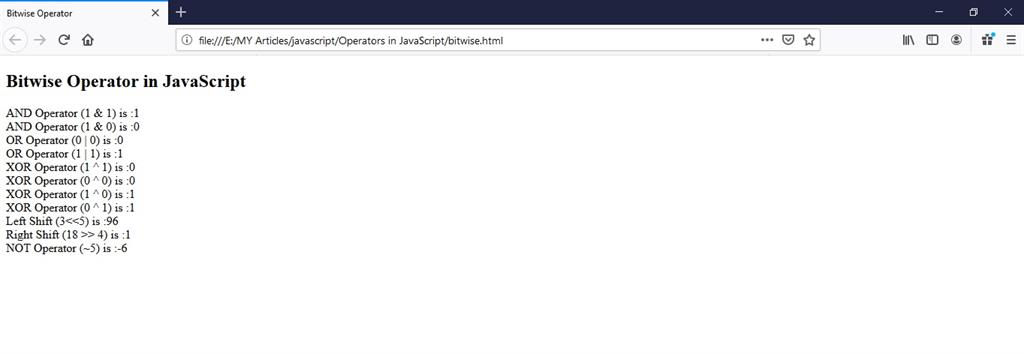## Assignment Operator

An assignment operator is one of the simplest operators used to assign a value to variable or constant. The right side could be a variable or constant, the left side of the operator must be a variable.

 Assignment Operator Description = Assigns the right-side value of the expression to the left side value of the expression. += Add the variables present on either side of the expression -= Subtract the variables present on either side of the expression *= Multiplication of the variables present on either side of the expression /= Divide the variables present on either side of the expression %= Modulus operation on the variables present on either side of the expression.

Example of assignment operators

Try it yourself:
1. <!DOCTYPE html>
2. <html>
4.     <title>Assignment Operator</title>
6. <body>
7.     <h2>Assignment operator in JavaScript</h2>
8.     <script type="text/javascript">
9.         var a=10;
10.         document.write("The value of a =",a);
11.         document.write("<br>");
12.         a=20; //the a value change 20
13.         document.write("The value of a =",a);// 20
14.         document.write("<br>");
15.         a+=20; //here the value of 20
16.         document.write("The value of a+ =",a);// 20+20 =40
17.         document.write("<br>");
18.         a-=10;
19.         document.write("The value of a- =",a);// 40-10 =30
20.         document.write("<br>");
21.         a*=10;
22.         document.write("The value of a* =",a);// 30*10 =300
23.         document.write("<br>");
24.         a/=10;
25.         document.write("The value of a/ =",a);// 300/10 =30
26.         document.write("<br>");
27.         a%=10;
28.         document.write("The value of a% =",a);// 30%10 =0
29.         document.write("<br>");
30.         //now the curent value of a is 0
31.     </script>
32. </body>
33. </html>
Output## Conditional Operators

Conditional operators are used to performing some conditional checks on expressions. The operator operates three expressions and contains symbols. Conditional operators also called ternary operators.

Syntax

<condition>? <value1>: <value 2>

Try it yourself:
1. <!DOCTYPE html>
2. <html>
4.     <title>Conditonal Operator </title>
6. <body>
7.     <h2>Conditional or ternary  operator in JavaScript</h2>
8.     <h2>Result</h2>
9.     Enter Name
10.     <input type="text" name="name1" id="n1">
11.     <br><br>
12.     Enter Mark
13.     <input type="number" name="mark" id="mrk">
14.     <br><br>
15.     <input type="submit" value="check" onclick="result();">
16.     <script type="text/javascript">
17.         function result() {
18.             var name = document.getElementById("n1").value;
19.             var mark = document.getElementById("mrk").value;
20.             (mark>=35)?document.write(name+"pass mark"):document.write(name+"Fail mark");// Conditional Operator
21.         }
22.     </script>
23. </body>
24. </html>
OutputTry it yourself: